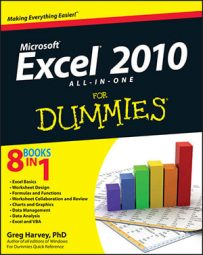##### Excel 2010 All-in-One For DummiesExcel 2010's IS information functions (as in ISBLANK, ISERR, ISNA, ISNUMBER, ISTEXT, and so on) are a large group of functions that perform essentially the same task. They evaluate a value or cell reference and return the logical TRUE or FALSE, depending on whether the value is or isn't the type for which the IS function tests. You'll find the Information functions by clicking the More Functions button on the Formulas tab of the Ribbon and in the Insert Function dialog box.

Excel offers nine built-in IS information functions. Each function requires only one argument — a value or cell reference that it analyzes:

• ISBLANK(value) evaluates whether the value or cell reference is empty.

• ISERR(value) evaluates whether the value or cell reference contains an Error value (other than #NA).

• ISERROR(value) evaluates whether the value or cell reference contains an Error value (including #NA).

• ISLOGICAL(value) evaluates whether the value or cell reference contains a logical TRUE or FALSE value.

• ISNA(value) evaluates whether the value or cell reference contains the special #NA Error value.

• ISNONTEXT(value) evaluates whether the value or cell reference contains any type of data type other than text.

• ISNUMBER(value) evaluates whether the value or cell reference is a numerical data type.

• ISREF(value) evaluates whether the value or cell reference is itself a cell reference.

• ISTEXT(value) evaluates whether the value or cell reference contains a text entry.

In addition to these nine IS functions, Excel adds two more, ISEVEN and ISODD, when you activate the Analysis ToolPak add-in. The ISEVEN function evaluates whether the number or reference to a cell containing a number is even, whereas the ISODD function evaluates whether it is odd. Both functions return the error value #VALUE if the cell reference isn't numerical.

Excel's IS functions evaluate the value argument as a whole. If, for example, the value in cell A1 were 40 Thieves,

`=ISTEXT(A1)`

would return TRUE, but

`=ISNUMBER(A1)`

would return FALSE.

Combining the IS functions with other functions can be especially useful in error handling; you can use these formulas to create your own, more informative error messages to replace Excel's. For example,

`=IF(AND(ISNUMBER(B2),B2>0),B1 * B2,"Please enter your tax rate in the appropriate box")`

This formula first evaluates the contents of cell B2. If cell B2 contains a number, and if that number is greater than zero, then the formula multiplies the values in cells B1 and B2 together (in this case, giving you the total tax due on the price listed in cell B1). In any other case — if B2 is empty, is a negative number, or contains text — this formula returns the phrase Please enter your tax rate in the appropriate box, which is much more informative than the zero amount, negative amount, or #VALUE error you would otherwise get if you just used the formula =B1 * B2.